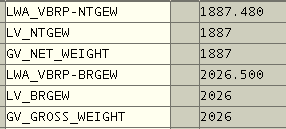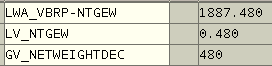﻿ Read Integer and Decimals of a Numeric Value in ABAP ProgrammingSAP ABAP Programming and HANA Database Tutorials Development resources, articles, tutorials, code samples, tools and downloads for SAP HANA and ABAP, HANA Database, SQLScript, SAP UI5, Screen Personas, Web Dynpro, Workflow

# Read Integer and Decimals of a Numeric Value in ABAP Programming

In ABAP programming, to read integer part of a numeric value and to read decimal part of a numeric value is very easy using numeric ABAP function TRUNC and FRAC function.

This ABAP tutorial will demonstrate how to read decimals of a given floating number using ABAP FRAC function. As well as reading decimals, developers will see how to read integer part of numeric values using ABAP TRUNC function. As one last step I'll concatenate integer part and decimals for a specific country using that locale's thousand separator and decimal separator character.

## Read Integers using ABAP TRUNC Function

Here is a sample case where ABAP developers can use TRUNC function to read integer part of numeric values in SAP systems. This ABAP example reads integer part of invoice items net and gross weight information from ABAP VBRP table BRGEW gross weight field and NTGEW net weight field for a given invoice number.This ABAP report will display 1887 as net weight and 2026 as gross weight value ignoring the decimals and only showing integer part of the item's weight data ntgew and brgew field values.

## Read Decimals using ABAP FRAC Function

ABAP programmers sometimes require to read decimals of numeric values for example for custom rounding in ABAP calculations. This ABAP program example will show how to read decimals of invoice item's weight data using ABAP FRAC function.Given above ABAP program will display 480 as decimal section of net weight of invoice item. If you use FRAC function for custom rounding, you can compare lv_ntgew with 0.500 for example within your ABAP report. If it is bigger or equal to limit value, you can increase the integer part by one. If it is less than limit value, you can just use the integer part of the numeric value for rounding off.

## Convert Numeric Value using Custom Thousands and Decimal Separator

After you have splitted integer value and decimal value of the floating numeric number in your ABAP report, developers can concatenate these two values using custom thousands and decimals separator. This text representation of numeric values might be a problem for different locales and especially if you are creating Microsoft Excel reports within your ABAP program.

In English thousand separator is "," and decimal separator is "."
For example, in Turkish the case is just the opposite. Thousands separator is "." and the decimal separator is "," in Turkish numeric formatting.

Here is a sample ABAP report to split numeric value into its integer and decimals part and concatenate back using custom separators.

Within the fn_convertTRnumericDisplay ABAP function, you can define your custom thousands and decimal separator as you wish for string representation of the numeric values.

SAP HANA and ABAP